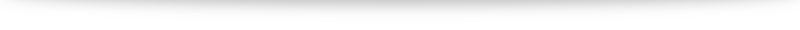# Chapter 1: Numbers, operations and axioms

### Numbers

Numbers are our first exposure to mathematics: yet what exactly a "number" is an interesting discussion I've had in philosophy classes. At least for "whole numbers", we typically find them pretty intuitive and it doesn't take a child much to be comfortable with numbers like "2" and "3" and learning how to operate on them via addition, "$2+3=5$" and multiplication "$2 \cdot 3 = 6$". The fact that there is a "two-ness" behind 2 cows, 2 dollars and 2 books is pretty remarkable we slow down and think about it. But that is a discussion for another day. Let us now look a few common sets of numbers we typically encounter:
• The natural numbers $\mathbb{N} = \{0, 1, 2, 3, \ldots \}$
• The integers $\mathbb{Z} = \{ \ldots, -2, -1, 0, 1, 2, \dots\}$
• The rational numbers $\mathbb{Q}$, numbers that can be expressed as $\frac{a}{b}$ where $a,b\in\mathbb{Z}, b \neq 0$
• The real numbers: visualized as a number line, including all the rational numbers and irrational numbers (e.g. $\sqrt{2}, \pi, e)$.
The fact that these set of numbers are useful to work with are encapsulated by the rules we want our operations, addition $+$ and multiplication $\cdot$ to follow and the axioms we want.

### Operations and axioms

To model how we think about numbers, addition and multiplication and how it works "in real life", we want them to follow certain rules. First (closure), for any numbers $a$ and $b$, we want $a+b$ and $a \cdot b$ to be numbers to. Also, these following should come as no surprise
1. Associativity: we want the order of repeated operations not to be important. $a + (b+c) = (a+c) + c, a \cdot (b \cdot c) = (a \cdot b) \cdot c$.
2. Commutativity: we want the order of operations not to matter. $a + b = b + a, a \cdot b = b \cdot a$
3. Distributive law: we want to know how addition and multiplication interacts. $a \cdot (b+c) = a \cdot b + a \cdot c$.
As an aside, commutative for multiplication is often not required when we study more abstract systems such as matrices in the study of abstract algebra. For our usual numbers that isn't a concern. The numbers $0$ and $1$ plays a special part in addition and multiplication respectively: they play the part as an "identity" element: $a + 0 = a$ and $a \cdot 1 = a$. Just the natural numbers satisfy all of the above axioms. Along with mathematical induction (chapter 2, which is implicit in the way the natural numbers are defined) these bring about the rich field of number theory. But things get more interesting (and allow for our study of calculus) when we require an "inverse" element, essentially setting up the field for the opposite operations of subtraction and division.
• Existence of additive inverse: for every number $a$, we have a number ($-a$) such that $a + (-a) = 0$.
• Existence of multiplicative inverse: for every number $a$, $a\neq 0$ we have a number ($a^{-1}$) such that $a \cdot a^{-1} = 1$.
Additive inverse brings about the integers, and multiplicative inverses bring about the rational numbers. The fact that 0 can not have an inverse will be investigated in a following post. Finally, we bring about the idea of "ordering" the numbers using the concept of an inequality. For any two numbers $a$ and $b$, one and only one of the following holds:
• $a=b,$
• $a < b,$ or
• $b < a$.
We want the inequality to have the following rules:
• If $a < b$ and $b < c$, then $a < c$
• If $a < b$, then for any $c$, $a+c < b+c$
• If $a < b$ and $0 < c$, then $ac < bc$
Just axioms alone (along with mathematical induction in Chapter 2) can lead us to a whole bunch of familiar techniques we have already internalized. We will explore them in solving some exercises in a subsequent post. We also note that just the rational numbers alone are sufficient to satisfy all the above axioms. But very quickly we realize that will preclude a solution to something "simple" like $x^2 = 2$. A proof that $\sqrt{2}$ is rational is presented in many other places: I will refer readers to Google if they have not seen it before. $x^2 = 2$ comes up pretty naturally from the study of geometry (the length of the hypotenuse in an isosceles right angled triangle with two sides of length 1) so real numbers are required for the study of subjects where we need a "measure". The construction of the reals is something I'm looking forward to at the end of the book.

### Modulus and the triangle inequality

The modulus/absolute value function (defined by $|a| = a$ if $a$ is positive or 0, and $|a| = -a$ if $a$ is negative), along with the triangle inequality $|a + b| \leq |a| + |b|$ comes up all the time in subsequent work so I will just mention that at the end of this post. The proof can be done by simply working through all the cases where $a$ and $b$ take different signs.